How to Learn in 24 Hours?The Rapid Learning Movie

 Need Help? M-F: 9am-5pm(PST): Toll-Free: (877) RAPID-10 US Direct: (714) 692-2900 Int'l: 001-714-692-2900 24/7 Online Technical Support: The Rapid Support Center Secure Online Order:Need Proof? Testimonials by Our Users

 Rapid Learning Courses: MCAT in 24 Hours (2015-16) USMLE in 24 Hours (Boards) Chemistry in 24 Hours Biology in 24 Hours Physics in 24 Hours Mathematics in 24 Hours Psychology in 24 Hours SAT in 24 Hours ACT in 24 Hours AP in 24 Hours CLEP in 24 Hours DAT in 24 Hours (Dental) OAT in 24 Hours (Optometry) PCAT in 24 Hours (Pharmacy) Nursing Entrance Exams Certification in 24 Hours eBook - Survival Kits Audiobooks (MP3)

 Tell-A-Friend: Have friends taking science and math courses too? Tell them about our rapid learning system.Home »  Mathematics »  Pre-Calculus

Graphs of Trigonometric Functions

 Topic Review on "Title": Periodic function: A periodic function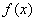must have the characteristic of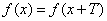where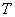is the period.  Horizontal shift of a function: The graph of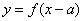is the graph of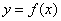shifted horizontally by a units.  Vertical stretching of a function: The graph of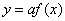is the graph ofstretched vertically by a units.  Horizontal shrinking of a function: The graph of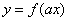is the graph ofshrunk horizontally by a units..  Reflection and vertical shift of a function: The graph of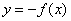is the reflection of the graphabout x-axis. The graph of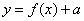is the graph ofshifted vertically by a units. Sinusoidal functions: Functions whose equations satisfy.  Amplitude: A nonnegative scalar measure of the magnitude of sinusoidal functions.  Period: For a sinusoidal function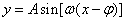the absolute value of is the period of the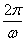sinusoidal function. Phase shift of a sinusoidal function: For a sinusoidal functionthe value of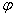is the phase shift of the sinusoidal function.

Rapid Study Kit for "Title":
 Flash Movie Flash Game Flash Card Core Concept Tutorial Problem Solving Drill Review Cheat Sheet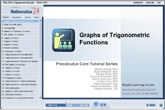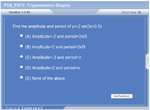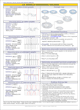"Title" Tutorial Summary : This tutorial is all about the graphs of the trigonometric functions.  First, the graphs of sine, cosine, tangent, cotangent, cosecant and secant functions are introduced with the help of examples. Next, equations for a sinusoidal graph are shown in examples with the sine and cosine functions. Properties of sinusoidal functions such as amplitude and period are introduced with graphs and tables. Next, the transformations of sinusoidal graphs are shown in the examples. The phase shift of a sinusoidal function is defined with a graphical illustration. There are two methodologies to obtain amplitude, period and phase shifts of trigonometric functions. Last, the tangent and the remaining trigonometric equations are defined in terms of their periodicity and amplitude.

 Tutorial Features: Specific Tutorial Features: • Animated diagrams showing thermal processes • Graphic organizers to illustrate concept relationships. Series Features: • Concept map showing inter-connections of new concepts in this tutorial and those previously introduced. • Definition slides introduce terms as they are needed. • Visual representation of concepts • Animated examples—worked out step by step • A concise summary is given at the conclusion of the tutorial.

 "Title" Topic List: The graphs of y=sin(x) Periodic functions Properties of sin(x) Graph transformations The graphs of y=cos(x) Properties of cos(x) Sinusoidal graphs Graphs of and Sinusoidal functions Amplitude of a sinusoidal function Period of a sinusoidal function Phase shift of a sinusoidal function The graphs of y=tan(x) Properties of tan(x) Graph transformations of tan(x) The graphs of y=cot(x) The graphs of y=csc(x) The graphs of y=sec(x)

See all 24 lessons in Pre-Calculus, including concept tutorials, problem drills and cheat sheets:
Teach Yourself Pre-Calculus Visually in 24 Hours